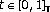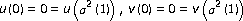Theory and Modern Applications

# Eigenvalue Problems for Systems of Nonlinear Boundary Value Problems on Time Scales

## Abstract

Values of λ are determined for which there exist positive solutions of the system of dynamic equations,,, for, satisfying the boundary conditions,, whereis a time scale. A Guo-Krasnosel'skii fixed point-theorem is applied.

## References

1. Agarwal RP, O'Regan D: Triple solutions to boundary value problems on time scales. Applied Mathematics Letters 2000,13(4):7–11. 10.1016/S0893-9659(99)00200-1

2. Anderson DR: Eigenvalue intervals for a second-order mixed-conditions problem on time scale. International Journal of Nonlinear Differential Equations 2002, 7: 97–104.

3. Anderson DR: Eigenvalue intervals for a two-point boundary value problem on a measure chain. Journal of Computational and Applied Mathematics 2002,141(1–2):57–64. 10.1016/S0377-0427(01)00435-6

4. Bohner M, Peterson A: Dynamic Equations on Time Scales: An Introduction with Applications. Birkhäuser, Boston, Mass, USA; 2001:x+358.

5. Chyan CJ, Davis JM, Henderson J, Yin WKC: Eigenvalue comparisons for differential equations on a measure chain. Electronic Journal of Differential Equations 1998, (35):7 pp..

6. Erbe LH, Peterson A: Positive solutions for a nonlinear differential equation on a measure chain. Mathematical and Computer Modelling 2000,32(5–6):571–585. 10.1016/S0895-7177(00)00154-0

7. He Z: Double positive solutions of boundary value problems for p -Laplacian dynamic equations on time scales. Applicable Analysis 2005,84(4):377–390. 10.1080/00036810500047956

8. Chyan CJ, Henderson J: Eigenvalue problems for nonlinear differential equations on a measure chain. Journal of Mathematical Analysis and Applications 2000,245(2):547–559. 10.1006/jmaa.2000.6781

9. Li W-T, Sun H-R: Multiple positive solutions for nonlinear dynamical systems on a measure chain. Journal of Computational and Applied Mathematics 2004,162(2):421–430. 10.1016/j.cam.2003.08.032

10. Sun H-R: Existence of positive solutions to second-order time scale systems. Computers & Mathematics with Applications 2005,49(1):131–145. 10.1016/j.camwa.2005.01.011

11. Erbe LH, Wang H: On the existence of positive solutions of ordinary differential equations. Proceedings of the American Mathematical Society 1994,120(3):743–748. 10.1090/S0002-9939-1994-1204373-9

12. Guo DJ, Lakshmikantham V: Nonlinear Problems in Abstract Cones, Notes and Reports in Mathematics in Science and Engineering. Volume 5. Academic Press, Boston, Mass, USA; 1988:viii+275.

13. Henderson J, Wang H: Positive solutions for nonlinear eigenvalue problems. Journal of Mathematical Analysis and Applications 1997,208(1):252–259. 10.1006/jmaa.1997.5334

14. Hu L, Wang L: Multiple positive solutions of boundary value problems for systems of nonlinear second-order differential equations. Journal of Mathematical Analysis and Applications 2007,335(2):1052–1060. 10.1016/j.jmaa.2006.11.031

15. Webb JRL: Positive solutions of some three point boundary value problems via fixed point index theory. Nonlinear Analysis: Theory, Methods & Applications 2001,47(7):4319–4332. 10.1016/S0362-546X(01)00547-8

16. Agarwal RP, O'Regan D, Wong PJY: Positive Solutions of Differential, Difference and Integral Equations. Kluwer Academic, Dordrecht, The Netherlands; 1999:xii+417.

17. Graef JR, Yang B: Boundary value problems for second order nonlinear ordinary differential equations. Communications in Applied Analysis 2002,6(2):273–288.

18. Infante G: Eigenvalues of some non-local boundary-value problems. Proceedings of the Edinburgh Mathematical Society 2003,46(1):75–86.

19. Infante G, Webb JRL: Loss of positivity in a nonlinear scalar heat equation. Nonlinear Differential Equations and Applications 2006,13(2):249–261. 10.1007/s00030-005-0039-y

20. Henderson J, Wang H: Nonlinear eigenvalue problems for quasilinear systems. Computers & Mathematics with Applications 2005,49(11–12):1941–1949. 10.1016/j.camwa.2003.08.015

21. Henderson J, Wang H: An eigenvalue problem for quasilinear systems. The Rocky Mountain Journal of Mathematics 2007,37(1):215–228. 10.1216/rmjm/1181069327

22. Ma R: Multiple nonnegative solutions of second-order systems of boundary value problems. Nonlinear Analysis: Theory, Methods & Applications 2000,42(6):1003–1010. 10.1016/S0362-546X(99)00152-2

23. Wang H: On the number of positive solutions of nonlinear systems. Journal of Mathematical Analysis and Applications 2003,281(1):287–306.

24. Zhou Y, Xu Y: Positive solutions of three-point boundary value problems for systems of nonlinear second order ordinary differential equations. Journal of Mathematical Analysis and Applications 2006,320(2):578–590. 10.1016/j.jmaa.2005.07.014

## Author information

Authors

### Corresponding author

Correspondence to M. Benchohra.

## Rights and permissions

Reprints and Permissions

Benchohra, M., Henderson, J. & Ntouyas, S.K. Eigenvalue Problems for Systems of Nonlinear Boundary Value Problems on Time Scales. Adv Differ Equ 2007, 031640 (2008). https://doi.org/10.1155/2007/31640

• Accepted:

• Published:

• DOI: https://doi.org/10.1155/2007/31640

### Keywords

• Differential Equation
• Partial Differential Equation
• Ordinary Differential Equation
• Functional Analysis
• Functional Equation